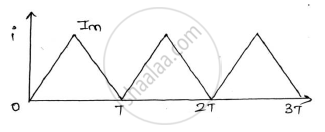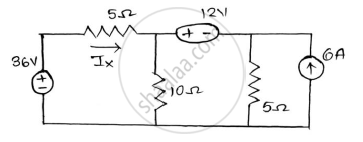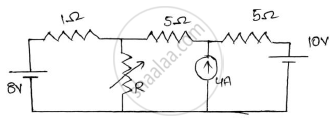Share

# Basic Electrical and Electronics Engineering CBCGS 2018-2019 BE Biomedical Engineering Semester 1 (FE First Year) Question Paper Solution

Basic Electrical and Electronics Engineering [CBCGS]
Date: December 2018

1) Question no. 1 is compulsory.
2) Answer any Three from the remaining five questions.

undefined
1
undefined
1.a

Find the RMS value of the waveform given below:-Concept: RMS and Average Value
Chapter:  A.C Circuits
undefined
1.b

State Norton’s theorem and draw the Norton’s equivalent circuit.

Concept: Norton’S Theorem
Chapter:  D.C. Circuits
undefined
1.c

In an R-L-C parallel circuit the current through the resistor, inductor(pure), and capacitor (pure) are 20A, 15A and 40A respectively. What is the current from the supply ?draw the phasor diagram.

Concept: R-L, R-C and R-LC parallel circuits
Chapter:  A.C Circuits
undefined
1.d

A balanced 3 phase star connected load consists of three coils each consisting of R=6Ω and 𝑿𝑳= 8Ω . Determine the line current , power factor when the load is connected across 400V, 50Hz, supply

Concept: Three Phase Current Generation
Chapter:  Three Phase Circuits
undefined
1.e

Briefly explain the classification of DC machine.

Concept: Construction and Classification of Dc Machines
Chapter:  DC Machines
undefined
1.f

Draw the phasor diagram of a single phase transformer when it is loaded with a lagging power factor load.

Concept: phasor diagrams of Single Phase Transformer
Chapter:  Single Phase Transformer
undefined
2
undefined
2.a

Prove that the average power taking by a pure capacitor fed with a sinusoidal ac supply in a circuit is zero.

Concept: Power and Power Factor
Chapter:  A.C Circuits
undefined
2.b

Using the mesh analysis find the mesh current in the direction shown and also find the voltage across A and B terminals.

Concept: Mesh Analysis
Chapter:  D.C. Circuits
undefined
3
undefined
3.a

A single phase transformer has 1000 turns on the primary and 200 turns on the secondary. The no load current is 3A at a power factor of 0.2 lag and the secondary current is 280A at a power factor of 0.8 lag. Neglect Rand X2 calculate (1) Magnetizing component and loss component of no load current. (2) primary current (3) input power factor .Draw phasor diagram showing all the currents.

Concept: Construction of Single Phase Transformer
Chapter:  Single Phase Transformer
undefined
3.b

Derive the formula for resonant frequency of the circuit with a pure capacitor in parallel with a coil having resistance and inductance. Find the expression for dynamic resistance of this parallel resonant circuit.

Concept: Series and Parallel Resonance
Chapter:  A.C Circuits
undefined
4
undefined
4.a

Find current IX using Superposition theoremConcept: Superposition Theorem
Chapter:  D.C. Circuits
undefined
4.b

A resistance and a capacitor connected in series across a 250V supply draws 5A at 50Hz. When frequency is increased to 60Hz, it draws 5.8A. Find the values of R & C. Also find active power and power factor in both cases.

Concept: AC Through Resistance
Chapter:  A.C Circuits
undefined
5
undefined
5.a

Find the node voltages 𝑽𝟏,𝑽𝟐 𝒂𝒏𝒅 𝑽𝟑 and current through 0.5Ω

Concept: Three Phase Voltage Genration
Chapter:  Three Phase Circuits
undefined
5.b

Describe the basic principle of operation of a single phase transformer and derive the emf equation.

Concept: Working Principle of Single Phase Transformer
Chapter:  Single Phase Transformer
undefined
6
undefined
6.a

Determine the value of R for maximum power transfer and find the value of maximum transfer.Concept: Maximum Power Transfer Theorem
Chapter:  D.C. Circuits
undefined
6.b

The OC and SC test data are given below for a single phase, 5KVA, 200V/400V, 50Hz transformer

 OC test from LV side 200V 1.25A 150w SC test from HV side 20V 12.5A 175w

Determine the following :-
1) Draw the equivalent circuit of the transformer referred to LV side.
2) At what load or KVA the transformer is to be operated for maximum efficiency?
3) Calculate the value of maximum efficiency.
4) Regulation of the transformer at full load 0.8power factor lagging.

Concept: OC and SC Test
Chapter:  Single Phase Transformer

#### Request Question Paper

If you dont find a question paper, kindly write to us

View All Requests

#### Submit Question Paper

Help us maintain new question papers on Shaalaa.com, so we can continue to help students

only jpg, png and pdf files

## University of Mumbai previous year question papers Semester 1 (FE First Year) Basic Electrical and Electronics Engineering with solutions 2018 - 2019

University of Mumbai Semester 1 (FE First Year) Basic Electrical and Electronics Engineering question paper solution is key to score more marks in final exams. Students who have used our past year paper solution have significantly improved in speed and boosted their confidence to solve any question in the examination. Our University of Mumbai Semester 1 (FE First Year) Basic Electrical and Electronics Engineering question paper 2019 serve as a catalyst to prepare for your Basic Electrical and Electronics Engineering board examination.
Previous year Question paper for University of Mumbai Semester 1 (FE First Year) Basic Electrical and Electronics Engineering-2019 is solved by experts. Solved question papers gives you the chance to check yourself after your mock test.
By referring the question paper Solutions for Basic Electrical and Electronics Engineering, you can scale your preparation level and work on your weak areas. It will also help the candidates in developing the time-management skills. Practice makes perfect, and there is no better way to practice than to attempt previous year question paper solutions of University of Mumbai Semester 1 (FE First Year).

How University of Mumbai Semester 1 (FE First Year) Question Paper solutions Help Students ?
• Question paper solutions for Basic Electrical and Electronics Engineering will helps students to prepare for exam.
• Question paper with answer will boost students confidence in exam time and also give you an idea About the important questions and topics to be prepared for the board exam.
• For finding solution of question papers no need to refer so multiple sources like textbook or guides.